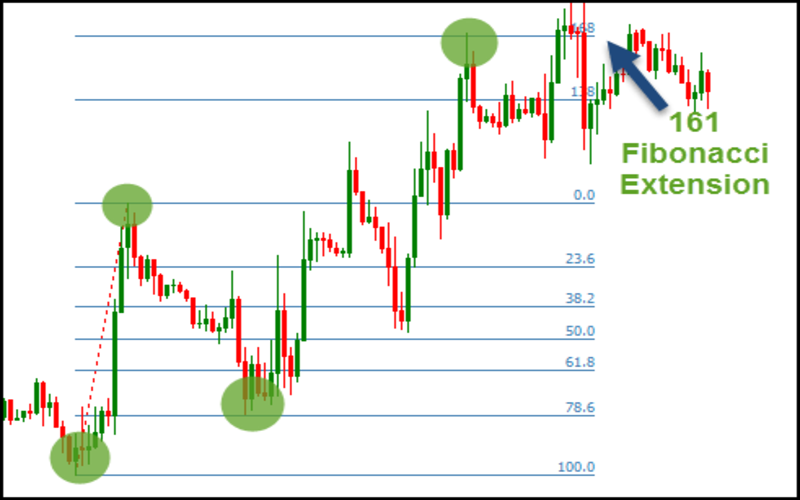Connect with us

Hi, what are you looking for?

DOGE0.070.84%SOL19.370.72%USDC1.000.01%BNB287.900.44%AVAX15.990.06%XLM0.080.37%

# Fibonacci Extensions: What They Are and How To Use ThemFile Photo: Fibonacci Extensions: What They Are and How To Use Them File Photo: Fibonacci Extensions: What They Are and How To Use Them

### What are Fibonacci extensions?

Fibonacci extensions can help traders set profit targets or predict market movement following a retreat. Price may revert at extension levels.

Fibonacci ratios (percentages) form these chart levels. Common Fibonacci extension levels include 61.8%, 100%, 161.8%, 200%, and 261.8%.

### Making Fibonacci Extensions

Galaxy formations, architecture, shells, storms, and flora use Fibonacci ratios. Thus, some traders feel these common ratios may be necessary in financial markets.

No formula exists for Fibonacci extensions. The trader selects three points when applying the indicator to a chart. The first point is the start of the move, the second is the end, and the third is the end of the retracement against that move. Extensions help predict price movements. The lines are created at percentages of the motion after selecting the three spots.

Chart extensions indicate price levels of potential relevance. These levels are based on percentage Fibonacci ratios and the amount of the price move the indicator is applied to.

### Calculating Fibonacci Retracement Levels

Calculate Fibonacci retracement levels by following these steps:

1. Multiply the difference between points one and two by any ratio, such as 1.618 or 0.618. This delivers a monetary amount.

2. If predicting a price increase, add the dollar amount above point three. If expecting a price drop, remove step one’s dollar amount from point three’s price.

As long as, the price goes from \$10 to \$20 to \$15, \$10 might be point one, \$20 point two, and \$15 point three. Above \$15, Fibonacci levels will be projected to show where the price may move next. If the price reduces, the indication must be redesigned to reflect point three’s reduced price.

If the price climbs from \$10 to \$20 at points one and two on the indicator, the 61.8% mark will be \$6.18 (0.618 x \$10) above point three. Point three is \$15. Thus, the 61.8% extension level is \$21.18 (\$15 + \$6.18). 100% is \$10 over point three for a \$25 extension ((1.0 x \$10) + 15).

The golden mean underpins the ratios. To understand this ratio, start a number string with zero and one, then add the preceding two numbers to get this:

0, 1, 1, 2, 3, 5, 8, 13, 21, 34, 55, 89, 144, 233, 377, 610, 987…

The Fibonacci extension levels come from this number string. Except for the first few digits, dividing one number by the previous number yields a ratio of 1.618, or 233 by 144. When dividing by the two remaining places, a number’s ratio approaches 2.618. When you divide by three to the left, the ratio is 4.236.

The critical Fibonacci extension levels are 23.6%, 38.2%, 50%, 61.8%, and 78.6%. Also standard: 100%, 161.8%, 200%, and 261.8%. Although not Fibonacci numbers, the 100% and 200% levels are essential since they predict a comparable move (or multiple of that move) to the price chart.

### What Do Fibonacci Extensions Say?

Fibonacci extensions can provide price goals or discover anticipated regions of support or resistance when the price moves into an area where other approaches fail.

Price may go to the next extension level after passing one. However, Fibonacci extensions may be interesting. The price may not halt or reverse at this level, but the surrounding region may matter. The price may swing close past or just shy of 1.618 before changing direction.

A long trader can utilize Fibonacci extension levels to predict where a stock may go after a new high. The same goes for short traders. Calculating Fibonacci extension levels might help traders set profit targets. Traders can then cover the position at that level.

Fibonacci extensions work in any market or period. Groups of Fibonacci levels usually suggest a price region that will affect the stock and traders’ decisions. When many extension levels from distinct price waves converge at one price, it may be a big deal.

### The Difference Between Fibonacci Extensions and Retracements

Extensions represent where the price will move after a pullback, while Fibonacci levels indicate depth. In other words, Fibonacci retracements measure trend pullbacks, while Fibonacci extensions measure trend impulse waves.

### Use of Fibonacci Extensions Limitations

Fibonacci extensions should not be used alone to purchase or sell stocks. Extensions and other signs or patterns should help investors set price objectives. Candlestick patterns and price activity can predict stock reversals at target prices.

Price may not reach or reverse an extension level. Even if it does, the critical Fibonacci extension level is unknown before a transaction. Price may quickly move through multiple levels or not reach any.

### Conclusion

• Because Fibonacci ratios are widespread in everyday life, some traders think they may be important in financial markets.
• No formula exists for Fibonacci extensions. They are drawn at three chart points to indicate potential price levels.
• Fibonacci extensions indicate the potential price movement after a retreat.
• Common Fibonacci extension levels include 61.8%, 100%, 161.8%, 200%, and 261.8%.
• Extension levels suggest important locations but should not be relied on alone.